# Two doctors

Doctor A will determine the correct diagnosis with a probability of 93% and doctor B with a probability of 79%. Calculate the probability of proper diagnosis if both doctors diagnose the patient.

p =  98.53 %

### Step-by-step explanation: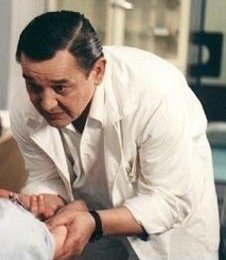Did you find an error or inaccuracy? Feel free to write us. Thank you!Tips to related online calculators
Would you like to compute count of combinations?

## Related math problems and questions:

• Doctors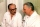The drug successfully treats 90% of cases. Calculate the probability that he will cure at least 18 patients out of 20?
• Alarm systems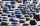What is the probability that at least one alarm system will signal the theft of a motor vehicle when the efficiency of the first system is 90% and of the independent second system 80%?
• MasteringThe student masters the subject matter for the exam in Czech to 98%, from Math to 86% and from Economics to 71%. What is the probability that he will fail from Math and from others will succeed?
• The manufacturer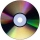The manufacturer found that 3% of the plates produced had a malfunction. Of the compliant, 75% are first and 25% second. What is the probability of producing first and second class plates?
• Probability - tickets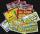What is the probability when you have 25 tickets in 5000 that you not wins the first (one) prize?
• Double probabilityThe probability of success of the planned action is 60%. What is the probability that we will achieve success at least once if this action is repeated twice?
• An aircraft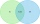An aircraft manufacturing company has submitted bids on two separate airline contracts, A and B. The company feels that it has a 70% chance of winning contract A and a 25% chance of winning contract B. Furthermore, it believes that winning contract A is i
• The vaccination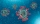The vaccination coverage of the population is 80%. Unvaccinated make up 60% of all infected. What percentage are unvaccinated more likely to be infected?
• Geography testsOn three 150-point geography tests, you earned grades of 88%, 94%, and 90%. The final test is worth 250 points. What percent do you need on the final to earn 93% of the total points on all tests?
• Dice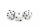How many times must throw the dice, the probability of throwing at least one six was greater than 90%?
• Probability of intersectionThree students have a probability of 0.7,0.5 and 0.4 to graduated from university respectively. What is the probability that at least one of them will be graduated?
• Left handedIt is known that 25% of the population is left-handed. What is the probability that there is a maximum of three left-handers at a seminar where there are 30 participants?
• Utopia IslandThe probability of disease A on the island of Utopia is 40%. The probability of occurrence among the men of this island, which make up 60% of all the population (the rest are women), is 50%. What is the probability of occurrence of A disease among women o
• AlcoholHow many 55% alcohol we need to pour into 14 liters 75% alcohol to get p3% alcohol? How many 65% alcohol we get?
• Lathe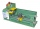95% of the components manufactured on the lathe comply with the standard, of which 80% of the components are first-class. How likely can we expect a manufactured part to be first class?
• The number 72The number 72 increase by 25%. By how much % will you have to reduce the number you created to get the number 72 again?
• ShootersIn army regiment are six shooters. The first shooter target hit with a probability of 49%, next with 75%, 41%, 20%, 34%, 63%. Calculate the probability of target hit when shooting all at once.